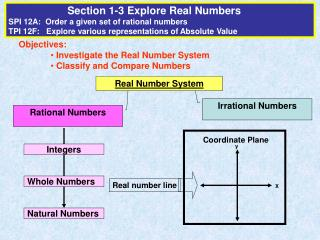DownloadDownload PresentationObjectives: Investigate the Real Number System Classify and Compare Numbers

# Objectives: Investigate the Real Number System Classify and Compare Numbers

Télécharger la présentation## Objectives: Investigate the Real Number System Classify and Compare Numbers

- - - - - - - - - - - - - - - - - - - - - - - - - - - E N D - - - - - - - - - - - - - - - - - - - - - - - - - - -
##### Presentation Transcript

1. Section 1-3 Explore Real NumbersSPI 12A: Order a given set of rational numbersTPI 12F: Explore various representations of Absolute Value • Objectives: • Investigate the Real Number System • Classify and Compare Numbers Real Number System Irrational Numbers Rational Numbers Coordinate Plane y Integers Whole Numbers Real number line X Natural Numbers

2. 1 2 3 4 5 6 7 8 9 10 11 0 1 2 3 4 5 6 7 8 9 10 11 -3 -2 -1 0 1 2 3 4 Real Number System Rational Numbers Irrational Numbers Any number that can be written in the form  where a and b are integers and b is not equal to 0. (Can be terminating, such as 6.27 .. Or .. Repeating like 8.222….) Any number that can not be written in the form of . (Nonrepeating & non-terminating) Natural Numbers 1, 2, 3, … Whole Numbers 0, 1, 2, 3, … Integers . . . -3, -2, -1, 0, 1, 2, 3, …

3. Name the set of numbers to which each number belongs a. - 3 b. 3.28 c.  d. . Rational, integer Rational Irrational Rational (its repeating)

4. Practice: Determine the set of numbers that is reasonable for a given situation. ALGEBRA 1 LESSON 1-3 1. Which set of numbers is most reasonable for displaying outdoor temperatures? integers 2. Which set of numbers is most reasonable for the number of students who will go on a class trip? whole 3. Which set of numbers is most reasonable to determine the height of a door? rational 4. Is the following statement true or false. If false, give a counterexample. “All negative numbers are integers.” False, because a negative number can be a fraction such as ½, which is not an integer.

5. -1 -1/2 -1/8 0 3/4 1 Comparing Numbers and Ordering Fractions Inequality: mathematical sentence that compares the value of 2 expressions. a < b a is less than b a > b a is greater than b a = b a is equal to b a  b a is less than or equal to b a  b a is greater than or equal to b a ≠ b a is not equal to b Compare a set of rational numbers using a number line and inequalities. Write -1/8, 1, ¾, -1/2, -1 from least to greatest. -1 < -1/2 < -1/8 < ¾ < 1

6. 3 4 7 12 5 8 – = –0.75 Write each fraction as a decimal. – = –0.583 – = –0.625 3 4 7 12 5 8 Order Fractions by Converting to Decimals Step 1: Convert fraction by dividing numerator by denominator Step 2: Compare the decimals: - the smaller number is the least (Pay attention to negative values)Step 3: Convert back to fractions and write in order Write – , – , and – , in order from least to greatest. -0.75 < -0.625 < -0.583 Write 1/12, -2/3 amd -5/8 in order from least to greatest. Step 1: Convert to decimal: 1/12 = .083 -2/3 = -.666666667 -5/8 = -.625 Step 2: Order decimals -.666666667 < -.625 < .083 Convert back to fraction -2/3 < -5/8 < 1/12

7. Absolute Values Opposites • Two numbers that have the same distance from zero on a number line but lie in opposite directions • Example: 1 and -1, 450 and -450, -1/2 and ½ Absolute Values • The distance of a number from zero on a number line • Written as a number enclosed by 2 vertical lines • Example: |3| and |-3| both have a value of 3

8. Modeling Absolute Value If you are at home and decide to take a ride on your motorcycle to your friends house, which is ten miles away. Your friend lives in the red house. How many miles did you travel away from your home? You and your friend decide to go to the store, the yellow house. How far is it from your friends house to the store? -10 0 10 Home

9. Practice Absolute Value Find each absolute value. a. |–2.5| b. |7| –2.5 is 2.5 units from 0 on a number line. 7 is 7 units from 0 on a number line. |–2.5| = 2.5 |7| = 7 c. |5 - 7| -2 is 2 units from 0 on a number line. |–2| = 2